c(a b) 表示 a b 序列在语料中出现的次数。 从这个计算公式我们可以看到一个缺点，就是当历史信息过长的时候，可能语料库里面没有完全一致的序列存在，随着历史信息的增加，数据稀疏的问题会比较显著，一个容易想到的做法是使用几个最近的历史词来代替整个历史词序列，这就引出了下一个话题：n-gram 语言模型。

### n-gram 语言模型与评价方法

n-gram 是指使用前 n-1 个词来估计当前（第 n 个）词，即 $p(w_i|w_1^{i-1})=p(w_i|w_{i-n+1}^{i-1})$。根据 n-gram 中 n 的值，我们有 unigram（$p(w_i)$）、bigram（$p(w_i|w_{i-1})$）、trigram（$p(w_i|w_{i-2}^{i-1})$） 等等。n-gram 中概率的计算方法也是通过计数来得到的，只不过和上面说的一样，也会有语料中不存在词序列，关于这个问题的解决方法我们在后面的平滑算法中提出来。

• WSJ 数据集，训练集有 38 million 词，测试集有 1.5 million 词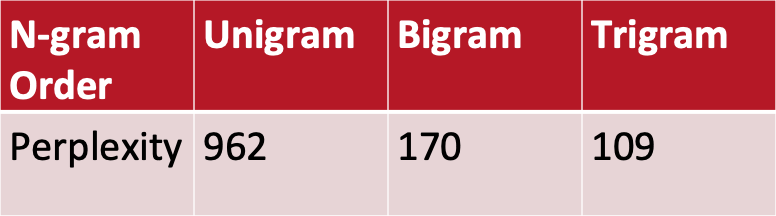### 平滑算法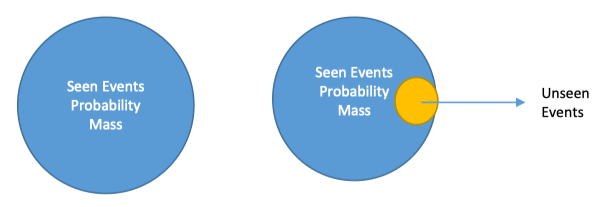#### 拉普拉斯平滑

# 词表大小为 7
my personal website is asr dot pub


ratio）。以 unigram 为例，我们将 $w_i$ 的计数记为 $c_i$，$P_{MLP}(w_i)=\frac{c_i}{N}$，$P_{laplace}(w_i)=\frac{c_i + 1}{N+V}$。调整计数的意义是描述平滑算法仅对 $P_{MLP}(w_i)$ 中分子的影响，所以我们需要将 $P_{MLP}$ 与 $P_{laplace}$ 中的分母先统一一下： $$\begin{split} P_{laplace}(w_i) &= \frac{C_i + 1}{N+V} \ &= \frac{\frac{(C_i + 1)*N}{N+V}}{N} \ \end{split}$$ 如上所示，$w_i$ 的计数由 $c_i$ 变成了 $\frac {(c_i + 1)*N}{N+V}$，所以调整计数为 $c^* = \frac {(c_i + 1)*N}{N+V}$，打折率 $d_c = \frac{c^*}{c_i}$，从公式可以看出打折率的意义是调整计数与原计数的比率 。

#### 古德图灵平滑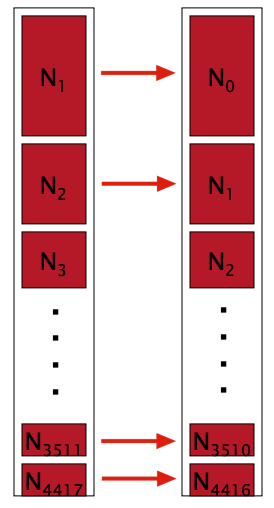# 一条文本
Sam I am I am Sam I do not eat

# 每个词出现的频率
I    3
sam  2
am   2
do   1
not  1
eat  1

# 各个频率的频数
N1 = 3
N2 = 2
N3 = 1


#### Jelinek–Mercer 平滑

• 使用 unigram 的 MLE 概率，或者其他的平滑概率
• 使用 zero-gram 的概率，也称为 uniform model，其值为 $P_{w_i}=\frac{1}{|V|}$，$|V|$ 是词表的大小

#### Witten-Bell 平滑

Witten–Bell 平滑开出出来的初衷是用于文本压缩的，可以认为它是 Jelinek–Mercer 平滑的一个特例。它的计算公式和上面的 Jelinek–Mercer 递归插值是一样的，只不过 WB 平滑在计算 $\lambda_{i-N+1}^{i-1}$ 时有它自己的方法。我们需要定义 $w_{i-N+1}^{i-1}$ 后面跟着的词的种类数，用符号 $N_{1+}(w_{i-N+1}^{i-1}·)$ 来表示，其正式的定义为： $$N_{1+}(w_{i-N+1}^{i-1}•) = |{ w_i:C(w_{i-N+1}^{i-1} w_i) > 0 }|$$ $N_{1+}$ 表示出现过一次以上的词序列种类数，• 表示任意的词都会被统计到。我们对 WB 平滑算法中的 $\lambda_{i-N+1}^{i-1}$ 赋值如下： $$1-\lambda_{i-N+1}^{i-1} = \frac {N_{1+}(w_{i-N+1}^{i-1}•)} { N_{1+}(w_{i-N+1}^{i-1}•) + \sum_{w_i} C(w_{i-N+1}^{i}) }$$ 所以我们带入上面 Jelinek–Mercer 递归公式中有： $$P_{WB}(w_i|w_{i-N+1}^{i-1}) = \frac { C(w_{i-N+1}^{i}) + N_{1+}(w_{i-N+1}^{i-1}•) P_{WB}(w_i|w_{i-N+2}^{i-1})} { N_{1+}(w_{i-N+1}^{i-1}•) + \sum_{w_i} C(w_{i-N+1}^{i}) }$$

#### 卡茨平滑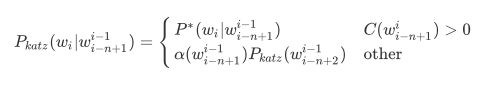$\alpha(w_{i-n+1}^{i-1})$ 被称为归一化因子，也就是训练好的语言模型 arpa 文件中最后一列回退概率。 $$\alpha(w_{i-n+1}^{i-1}) = \frac {1-\sum_{w_i:c(w_{i-n+1}^{i})>0}P_{GT}^*(w_i|w_{i-n+1}^{i-1})} {1-\sum_{w_i:c(w_{i-n+1}^{i})>0}P_{GT}^*(w_i|w_{i-n+2}^{i-1})}$$ $w_i:C(w_{i-n+1}^{i})$ 所表达的意义为：词序列 $w^{i-1}_{i-N+1}$ 是固定的， $w_i$ 是任意的。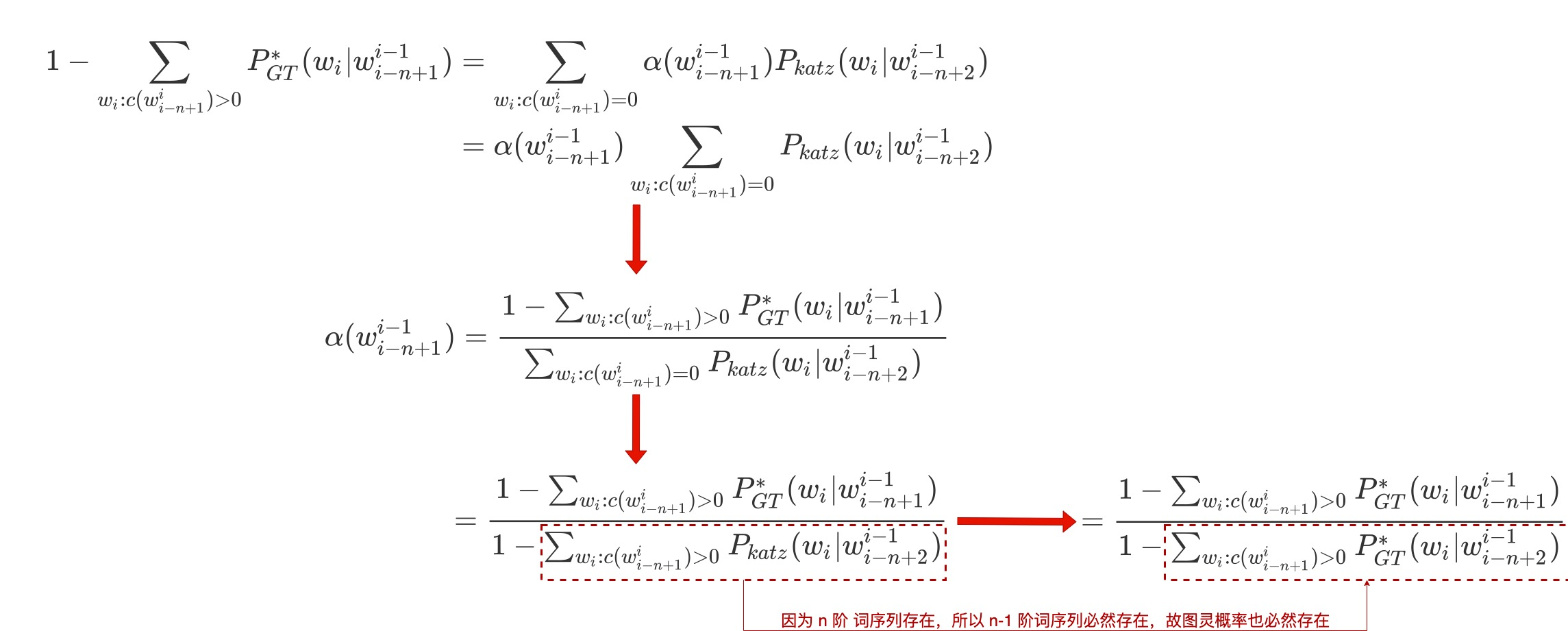#### 克奈瑟-内平滑

Church 和 Gale（1991）对具有 2200 万词的 AP Newswire 语料进行 Good-turing 打折计算后得到如下表格：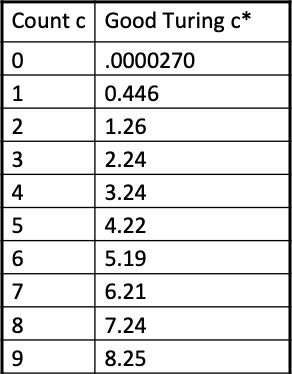### 参考文章

wiki n-gram

Dan Jurafsky - Language Model

Introduction to N-grams — [ NLP || Dan Jurafsky || Stanford University ]

Lecture 18 — Good Turing Smoothing — [ NLP || Dan Jurafsky || Stanford University ]

Kneser-Ney Smoothing

All Our N-gram are Belong to You

An empirical study of smoothing techniques for language modeling

4. Part 3: Implement Witten-Bell smoothing

Jelinek-Mercer smoothing

A Maximum Likelihood Approach to Continuous Speech Recognition